## Table of ContentOpen Access

ARTICLE

# Stability Scrutinization of Agrawal Axisymmetric Flow of Nanofluid through a Permeable Moving Disk Due to Renewable Solar Radiation with Smoluchowski Temperature and Maxwell Velocity Slip Boundary Conditions

Umair Khan1,2, Aurang Zaib3, Anuar Ishak1, Iskandar Waini4, El-Sayed M. Sherif5, Dumitru Baleanu6,7,8,*

1 Department of Mathematical Sciences, Faculty of Science and Technology, Universiti Kebangsaan Malaysia, Bangi, 43600, Malaysia
2 Department of Mathematics and Social Sciences, Sukkur IBA University, Sukkur, 65200, Pakistan
3 Department of Mathematical Sciences, Federal Urdu University of Arts, Science & Technology, Karachi, 75300, Pakistan
4 Fakulti Teknologi Kejuruteraan Mekanikal dan Pembuatan, Universiti Teknikal Malaysia Melaka, Melaka, 76100, Malaysia
5 Mechanical Engineering Department, College of Engineering, King Saud University, Riyadh, 11423, Saudi Arabia
6 Department of Mathematics, Cankaya University, Ankara, 06790, Turkey
7 Institute of Space Sciences, Magurele, 077125, Romania
8 Department of Medical Research, China Medical University Hospital, China Medical University, Taichung, 40447, Taiwan

* Corresponding Author: Dumitru Baleanu. Email:Computer Modeling in Engineering & Sciences 2023, 134(2), 1371-1392. https://doi.org/10.32604/cmes.2022.020911

## Abstract

The utilization of solar energy is essential to all living things since the beginning of time. In addition to being a constant source of energy, solar energy (SE) can also be used to generate heat and electricity. Recent technology enables to convert the solar energy into electricity by using thermal solar heat. Solar energy is perhaps the most easily accessible and plentiful source of sustainable energy. Copper-based nanofluid has been considered as a method to improve solar collector performance by absorbing incoming solar energy directly. The goal of this research is to explore theoretically the Agrawal axisymmetric flow induced by Cu-water nanofluid over a moving permeable disk caused by solar energy. Moreover, the impacts of Maxwell velocity and Smoluchowski temperature slip are incorporated to discuss the fine points of nanofluid flow and characteristics of heat transfer. The primary partial differential equations are transformed to similarity equations by employing similarity variables and then utilizing bvp4c to resolve the set of equations numerically. The current numerical approach can produce double solutions by providing suitable initial guesses. In addition, the results revealed that the impact of solar collector efficiency enhances significantly due to nanoparticle volume fraction. The suction parameter delays the boundary layer separation. Moreover, stability analysis is performed and is found that the upper solution is stable and physically trustworthy while the lower one is unstable.

## Keywords

Nomenclature

 T∞ Constant ambient temperature (K) b Constant parameter having units (m.s)−1 (ra,θa,za) Cylindrical coordinate system (m) Tw Constant surface temperature (K) wA Constant mass flux velocity (m/s) G(ξ) Dimensionless velocity stream function ue,ve,we Free-stream velocities (m/s) Nura Local Nusselt number Rera Local Reynolds number Pa Pressure (kg/m.s2) Pr Prandtl number Nr Radiation parameter qr Radiative heat flux uw Surface velocity of the disk (m/s) fw Suction parameter Cfra Skin friction coefficient along the radial direction cp Specific heat at constant pressure (J/kg.K) k Thermal conductivity (W/(m. K)) Ta Temperature of the fluid (K) (ua,va,wa) Velocity components (m/s) Greek symbols μ Absolute viscosity (N.s/m2) λa Coefficient of the main free path ρ Density (kg/m3) S(ξ) Dimensionless temperature Γ Eigenvalue υf Kinematic viscosity (m2/s) ka Mean absorption constant Σ New dimensionless variable ξ Pseudo-similarity variable βA Stretching/Shrinking parameter γA Specific heat ratio σa Stefan-Boltzmann constant (W/(m2.K4)) φ Solid nanoparticle volume fraction σTA Thermal accommodation coefficient αf Thermal diffusivity (m2/s) δB Temperature slip parameter σw Tangential momentum accommodation coefficient δA Velocity slip parameter Acronyms bvp4c Boundary value problem of the fourth-order BCs Boundary conditions Cu Copper HS Human society ICs Initial conditions LBS Lower branch solution ODEs Ordinary differential equations PDEs Partial differential equations SE Solar energy SPF Stagnation point flow 2D, 3D Two and three-dimensional UBS Upper branch solution H2O Water Subscripts ∞ Far-field condition nf Nanofluid s Solid nanoparticles f Working base fluid w Wall boundary condition Superscript ʹ Derivative with respect to ξ

1  Introduction

The slip boundary condition is defined as the module of tangential speed at the body surface that is proportional to the wall gradients (shear stress). After establishing a link between slip rate and shear stress, Navier  coined the term slip boundary condition. Maxwell  gave the most basic enlightenment for the momentum slip, which is based on a momentum gradient accommodation exerting normal to the surface as well as the creep term. Later, Smoluchowski  goes on to illuminate the concept of slip temperature. Ramya et al.  inspected the impacts of momentum and thermal slips on the dynamics of fluid flow scattered in nanomaterials. The rates of heat and mass transfer are reduced as the thermal slip parameter is increased. Khashi et al.  elaborated the impressions of slip and CBCs on a 3D flow comprising two dissimilar kinds of nanomaterials over a stretching/shrinking surface. The stability analysis has been performed to validate the stable solution. Jünemann et al.  utilized the Smoluchowski temperature boundary as well as Maxwell slip condition to stimulate the behavior of operation of screw machines. Recently, Sajid et al.  developed a 3D model subject to Sutterby rotating fluid flow in an expandable slippery surface with Smoluchowski temperature and Maxwell slip conditions. They discovered that in the case of a constant reaction rate, the thermal radiation parameter improves the heat transfer by 17.2 percent and the mass transfer by 62.1 percent.

A rigid or stiff body moving via a fluid (i.e., automobile, sports ball, missile, space travel vehicle), or in the recovery of oil in the industry (the extraction of crude oil from a lubricant ground is accomplished through gas equivalently or injection), a stagnation point signifies as an exterior flow encroaches in a stationary-point on the exterior of a submerged body within a flow, where the speed of the object is zero at the surface of the submerged object. Hiemenz  was the first to precisely analyze the classic problem of 2D stagnation-point flows (SPFs). As a result, the flow guided perpendicular to a flat infinite plate has an exact solution. The axisymmetric flow (AF) near a stagnation point (SP) from a plate was investigated by Homann . Ramya et al.  and Howarth  extended the 2D flow and axisymmetric flow to the 3D flow case using the approximation of the boundary layer in the path normal to the plane surface. In addition, Davey  developed an innovative axisymmetric SPF and presented an analytic solution to the Navier–Stokes equations by using spherical coordinates. On the contrary to the Agrawal  outer irrotational flow, this flow is rotational in the far-field. Weidman  has prolonged Agrawal’s work toward the situation of SP rotational flow impingement on a stretchable radially surface. Weidman [30,31] analyzed the AGAF near a SP from a respective stretched and flat sheet. Recently, Roşca et al.  investigated the viscous fluid flow impinging towards a stretching/shrinking sheet in a nanofluid and presented double solutions.

The axisymmetric flow past a permeable moving continuous disk in the existence of Maxwell and Smoluchowski slip conditions after being inspired by the literature mentioned aboveis simulated in this paper. In the existence of radiative solar energy, the thermal impartiality of the entire model is developed. The copper nanoparticles are used to simulate a flow with water as the base medium. There are very few publications dealing with axisymmetric flow induced by nanofluid flow past a stretching/shrinking sheet influenced by solar radiation. As a result, our novel exploration into the influence of radiative solar energy as well as slips interactions will help engineers implement nanofluids positively and effectively. The multiple solutions of the considered model are also one of the main interests of this work for the phenomena of shrinking and stretching disk. Therefore, stability analysis is also implemented to check which result is physically realizable.

2  Mathematical Modeling of the Problem

Consider the characteristics of heat transfer analysis and steady Agrawal axisymmetric SPF of a copper water-based nanofluid through a porous moving disk in the presence of solar energy radiation as shown schematically in Fig. 1. The flow configuration of the model is articulated in the form of cylindrical coordinates (ra,θa,za) measured in the radial, azimuthal and axial directions respectively, along with accompanying velocity modules (ua,va,wa). The AGF is exclusively symmetric to the raθaplane and likewise axisymmetric to the coordinate zaaxis, i.e., the change with respect to the coordinate θa is completely ignored. The surface is positioned at za=0, and the upper half-plane is the essential domain of the fluid flow. Further presume that the variable far field or ambient velocity is emphasized via ue(ra,za)=2braza and we(ra,za)=2bza2, where b corresponds the arbitrary factor evaluating the magnitude of the AGF having dimensions (LT)1, see Weidman . In addition, the wall variable velocity is highlighted via uw=b2/3υf1/3ra along with the requisite posited Maxwell slip velocity  having slip length 2σwσwλauaza have been employed to examine the momentum slip effect while the transparent constant mass flux is equal to wA, where wA<0 is for the case of suction and wA>0 is for the case of blowing. The Smoluchowski temperature slip  and the wall constant temperature is designated via 2σTAσTA(2γAγA+1)λaPrTaza and Tw, respectively, while the free-stream constant temperature is denoted via T by means of the phenomenon Tw>T. The surroundings and the Newtonian absorber are both kept at a constant temperature T, far away from the moving disk surface. Furthermore, an incoming radiation flux of intensity qr is received by the non-reflective, absorbent, preferably transparent movable disk. Rendering to Fathalah et al. , this qr penetrates the moving disk surface and is absorbed in a neighboring fluid with an absorption coefficient. Heat is transported from the disk surface to the surroundings as the absorbing nanoliquid and the moving disk surface are heated by SE. Also, solar radiation is a collimated beam that is perpendicular to the surface of the disk. As stated before, the regular (viscous) fluid is supposed to have heat absorption properties. However, the thermophysical properties of the working fluid and the nanoparticle are taken to be constant. Due to the significant impression of the aforesaid assumptions of the problem, the leading constitutive equations in terms of PDEs can be simply and written as Eqs. (1)(4) (see Weidman ):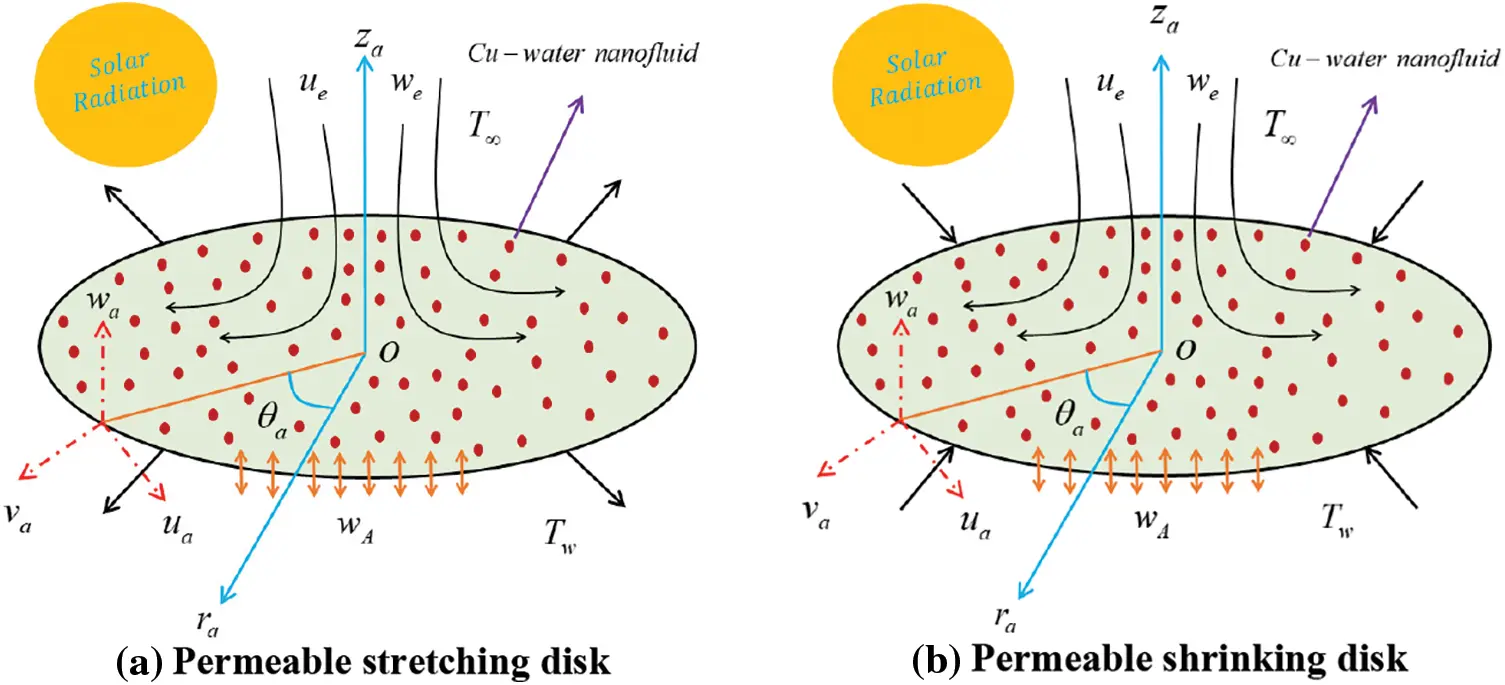Figure 1: Physical model of the Agrawal flow problem

1ra(raua)ra+waza=0,(1)

uauara+wauaza=1ρnfPara+μnfρnf(2uara2uara2+1rauara+2uaza2),(2)

uawara+wawaza=1ρnfPaza+μnfρnf(2wara2+1rawara+2waza2),(3)

uaTara+waTaza=knf(ρcp)nf(2Tara2+1raTara+2Taza2)1(ρcp)nfqrza,(4)

along with subject to the boundary conditions in Eq. (5)

ua=βAuw+2σwσwλauaza,wa=wA,Ta=Tw+2σTAσTA(2γAγA+1)λaPrTaza at za=0,uazaueza,TaTasza.}(5)

Here, wa and ua are the velocity components along za and ra axes, σw corresponds the coefficient of tangential momentum accommodation, Pa is the pressure, σTA corresponds the coefficient of thermal accommodation, λa corresponds the main free path coefficient, Ta corresponds the temperature, γA corresponds the specific heat ratio coefficient and βA corresponds the dimensionless shrinking/stretching constraint with βA<0 for shrinking, βA>0 for stretching, and βA=0 for the motionless disk.

In addition, the thermo-physical expressions of the copper-water based nanofluid are set by  in Eq. (6):

{μnfμf=(1φ)2.5,ρnfρf=φ(ρsρf)+(1φ),knfkf=(ks+2kf)2φ(kfks)(ks+2kf)+φ(kfks),(ρcp)nf(ρcp)f=φ((ρcp)s(ρcp)f)+(1φ),(6)

where φ namely resembles to the nanoparticles volume fraction which is equal to the mixtures of copper nanoparticle (Cu) and the regular (viscous) fluid (H2O). Additionally, kf, ρf, μf and (ρcp)f indicate the thermal conductivity, density, dynamic viscosity, and the specific heat capacity at a constant pressure of the regular (viscous) fluid, respectively, while ks, ρs and (ρcp)s are the respective quantities of the solid nanoparticles. Moreover, the subscripts s and f are classified as the solid nanoparticles and the base fluid, respectively. The experimental thermophysical characteristics of the regular pure liquid (water) and copper (Cu) nanoparticles are depicted in Table 1.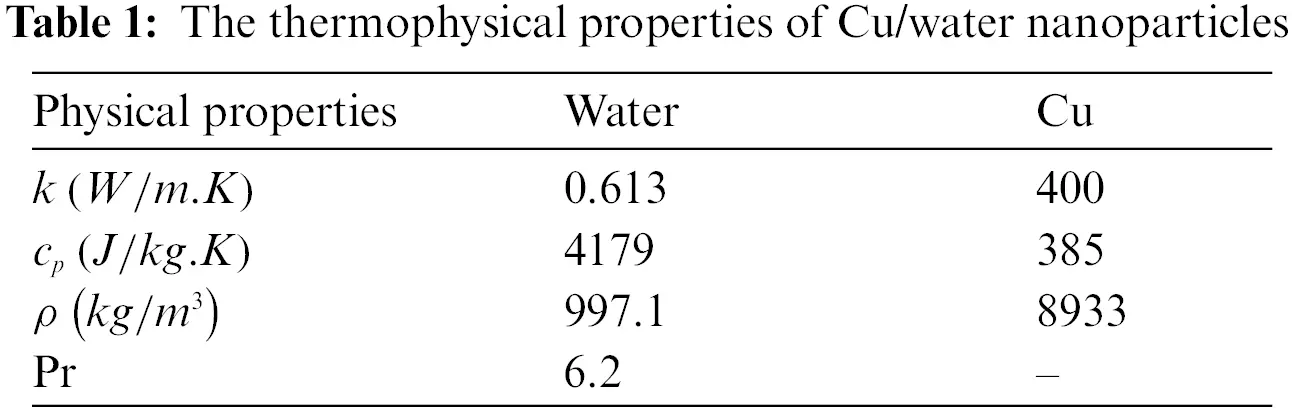Furthermore, the last term on the right-hand side of Eq. (4) is incorporated specially to demarcate the radiative heat flux qr. Also, given an optically thick fluid (nanofluid) layer, one can express the simplified version of the qr using the Rosseland approximation can be as Eq. (7) (see Hayat et al. ):

qr=4σa3kaTa4za,(7)

where σa and ka signify the Stefan Boltzmann and the mean absorption constants. Using Taylor series approximation centered around the point T, the term Ta4 can be simplified as Ta44T3Ta3T4, by neglecting the second and higher order power terms.

For making the system of equations dimensionless, the following similarity variables are employed in Eq. (8) (see Weidman ):

ua(ra,za)=b2/3υf1/3raG(ξ),ξ=(b/υf)1/3za,S(ξ)=TaTTwT,wa(ra,za)=2b1/3υf2/3G(ξ),(8)

where primes denote the differentiation w.r.t ξ and the last term of Eq. (8) leads to yield:

wA=2b1/3υf2/3fw.(9)

Here, fw=G(0) divulges the transparent mass flux dimensionless constraint with fw>0 and fw<0 resemble to blowing and suction, respectively, while fw=0 indicates the surface of the impermeable disk.

Now the similarity transformations are plugged into Eqs. (2) to (4), where Eq. (1) is identically satisfied and the rest of the equations are transformed to the following similarity ODEs:

μnf/μfρnf/ρfG+2GGG2=0,(10)

1Pr(ρcp)nf/(ρcp)f(knf/kf+43Nr)S+2GS=0,(11)

with boundary conditions:

{G(0)=fw,G(0)=βA+δAG(0),S(0)=1+δBS(0)G(ξ)2,S(ξ)0asξ.(12)

The dimensionless parameters in the aforesaid equations include the velocity slip parameter δA=(2σw/σw)λa(b/υf)1/3, the Prandtl number Pr=υf/αf, the thermal slip factor δB=(2σTA/σTA)(2γA/γA+1)λa/Pr(b/υf)1/3 and the radiation parameter Nr=(4σaT3)/kakf.

The quantities of engineering practical importance are the friction factor Cfra in the radial direction and the Nusselt number Nura, which are given as:

Cfra=μnfρfuw2(uaza)|za=0,Nura=rakf(TwT)(knf(Taza)+(qr)w)|za=0.(13)

Using similarity transformations in the above Eq. (13), we get Eq. (14):

Rera1/2Cfra=μnfμfG(0),Rera1/2Nura=(knfkf+43Nr)S(0).(14)

where Rera=uwraυf corresponds to the local Reynolds number.

3  Temporal Stability Analysis

In this segment, we study the stability of the multiple (upper and lower) solutions as time evolves. Merkin  was the first researcher to introduce the analysis of this technique, later; it was followed by Weidman et al. . For the measured working procedure, initially, we start from the new variables as follows:

ua=b2/3υf1/3raG(ξ,Σ)ξ,ξ=(b/υf)1/3za,S(ξ,Σ)=TaTTwT,wa=2b1/3υf2/3G(ξ,Σ).(15)

where Σ=b2/3υf1/3ta symbolizes the new dimensionless time. Further, executing the above Eq. (15) into the time-dependent or unsteady form of the requisite governing Eqs. (2) to (4), which can take place the form as:

μnf/μfρnf/ρf3Gξ3+2G2Gξ2(Gξ)22GξΣ=0,(16)

1Pr(ρcp)nf/(ρcp)f(knf/kf+43Nr)2Sξ2+2GSξSΣ=0,(17)

subject to the appropriate BCs:

{G(0,Σ)ξ=βA+δAG(0,Σ)ξ2,G(0,Σ)=fw,S(0,Σ)=1+δBS(0,Σ)ξ2G(ξ,Σ)ξ22,S(ξ,Σ)0 asξ.(18)

According to Weidman et al. , the time-independent outcome G=G0 and S=S0 of the similarity Eqs. (10) to (12) are perturbed as follows:

G(ξ,Σ)=G0(ξ)+eΓΣg(ξ),S(ξ,Σ)=S0(ξ)+eΓΣs(ξ),(19)

where the functions g(ξ) and s(ξ) in Eq. (19) are relatively small compared to G0(ξ) and S0(ξ). The stability of the outcomes is determined by the sign (positive or negative) of the eigenvalue Γ. Therefore, exercising Eq. (19) into the above Eqs. (16) and (17) which can take place the form as:

μnf/μfρnf/ρfg+2(G0g+G0gG0g)+Γg=0,(20)

1Pr(ρcp)nf/(ρcp)f(knf/kf+43Nr)s+2(G0s+S0g)+Γs=0,(21)

along with BCs:

g(0)=0,g(0)=δAg(0),s(0)=δBs(0)g(ξ)0, s(ξ)0 asξ.(22)

The corresponding positive or negative values of Γ in the above Eqs. (20) and (21) are found by setting the value of g(0) or s(0). Without loss of generality, we set g(0)=2 and solve the set of coupled Eqs. (20) to (22) to find the eigenvalues Γ.

4  Numerical Technique

The set of similarity Eqs. (10) and (11) with appropriate BCs (12) were cracked computationally by employing the bvp4c package available in MATLAB. The working procedure of the considered bvp4c scheme is described in detail by Shampine et al.  as well as Khan et al. . The recognized bvp4c approach was also built on a well-known finite difference approach that used the Lobatto IIIA collocation formula to construct a C1-continuous result. The syntax of the bvp4c solver is demarcated by “Sol = bvp4c (@OdeBVP, @OdeBC, Solinit, Options)”. Moreover, to start working on the employed technique, the system of second and third order equations is changed to the required first order by introducing the latest notations and rewriting them as follows:

G=Z1,G=Z2,G=Z3,S=Z4,S=Z5.(23)

Now to utilize the aforementioned Eq. (23) in the dimensionless set of Eqs. (10)(12) to derive the set of first-order ODEs, which has the form:

ddξ(Z1Z2Z3Z4Z5)=(Z2Z3ρnf/ρfμnf/μf(Z222Z1Z3)Z52Pr(ρcp)nf/(ρcp)f(knf/kf+(4Nr/3))Z1Z5).(24)

To treat the above set of differential equations as an initial boundary value problem, the following unknown and known initial conditions are considered:

Z1(0)=fw,Z2(0)=βA+δAZ3(0),Z3(0)=B1,Z4(0)=1+δBZ5(0),Z5(0)=B2.(25)

Moreover, the above-mentioned constants B1 and B2 are guessed during the bvp4c numerical execution by utilizing an operational shooting algorithm as explained practically by Khan et al. , in such a way that the executed iterative process is repeated multiply until satisfying all the following boundary conditions at once:

Z3(ξ)2,Z4(ξ)0asξ.(26)

Two alternative initial guesses for the values of the skin friction coefficient and the heat transfer rate are used to investigate the double (upper and lower) solutions. To get the preferred outcomes, the suitable starting estimate and thickness of the boundary layer ξ must be selected based on the parameters implemented. To authenticate the considered numerical scheme, the values of G(0) are compared with the case that has been reported in Weidman  for regular (viscous) fluid (φ=0) with several values of βA when fw=0 and δA=0 as presented in Table 2, which displays a satisfactory agreement. From this comparison, it can be noticed that the existing results for upper and lower branch solutions are usable and consistent. Therefore, we can conclude that the generated algorithm for the numerically investigating Agrawal (Cu-water) nanofluid flow can be used with great confident.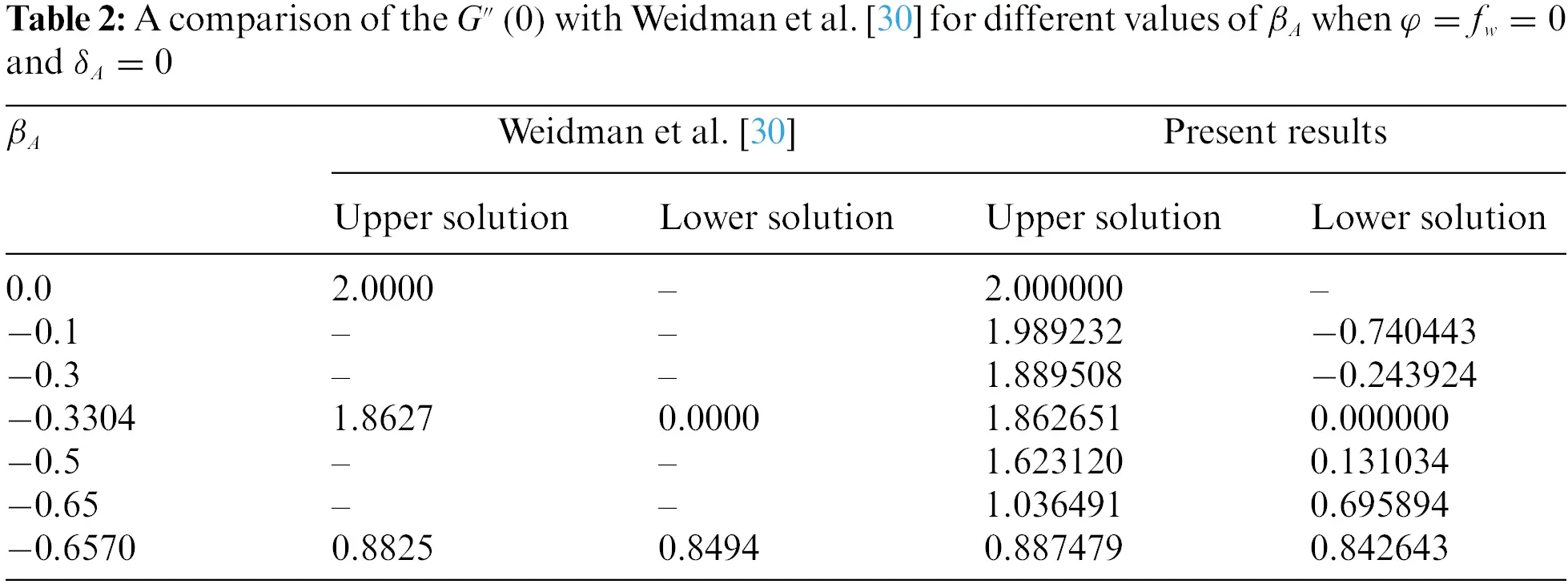5  Analysis of Results and Discussion

The present work aims to investigate the influence of the distinguished parameters such as the moving disk parameter βA, the velocity slip parameter δA, the thermal slip parameter δB, the radiation parameter Nr, the mass suction parameter fw, and the nanoparticles volume fraction φ on the reduced heat transfer, temperature profile and reduced shear stress of the (Cu-water) nanofluid for the double results as revealed in Figs. 2 to 9. Moreover, the computational values of the reduced shear stress and heat transfer of the (Cu-water) nanofluid for the upper branch solution (UBS) and lower branch solution (LBS) with the influence of the several influential parameters when λ=1.5 (shrinking sheet) and Pr=6.2 are elucidated in Tables 3 and 4, respectively. The numerical and graphical behavior of the eigenvalues Γ for several values of βA is presented in Table 5 and Fig. 10, respectively. For the aims of computation, the fix values of the embedded distinguished factors are written as follows: βA=1.4, fw=0.5, δA=0.05, δB=0.5, Nr=1.5 and φ=0.03. Therefore, the UBS and LBS throughout the graphs are signified by the black solid and dash lines, respectively, while the small solid red, blue, and grey balls are called the bifurcation point. The stable solutions are found on the UB, while unstable solutions are found on the LB. The stable solutions are of physical interest because they may be tested experimentally or understood practically.Figure 2: Variations of Rera1/2Cfra against βA for dissimilar choices of fw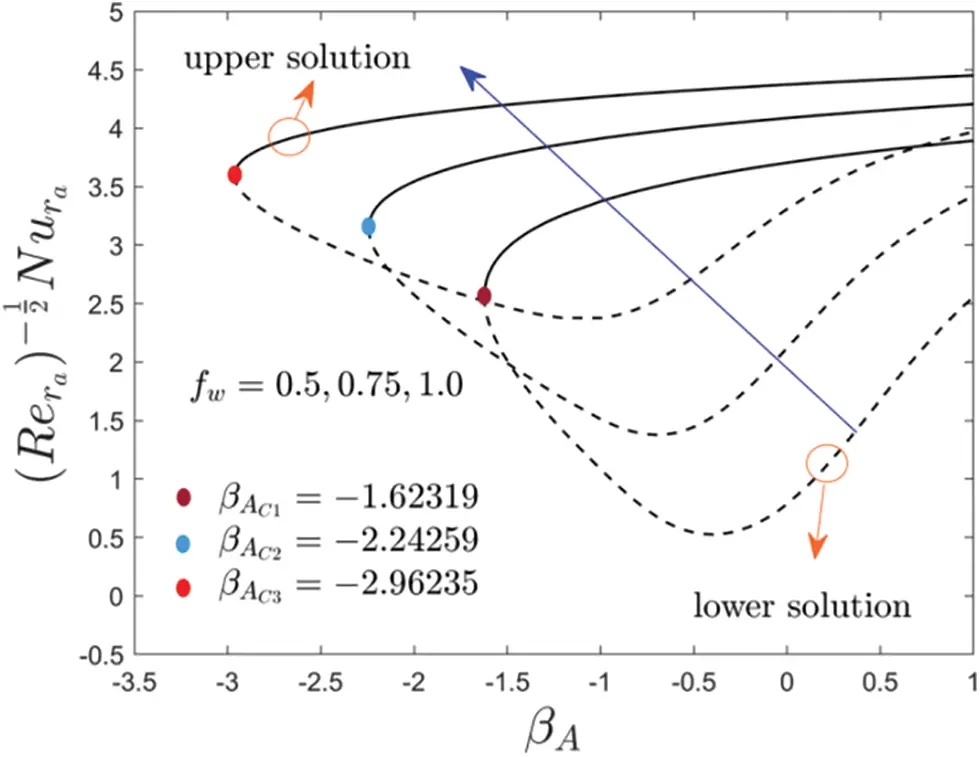Figure 3: Deviations of Rera1/2Nura against βA for several values of fw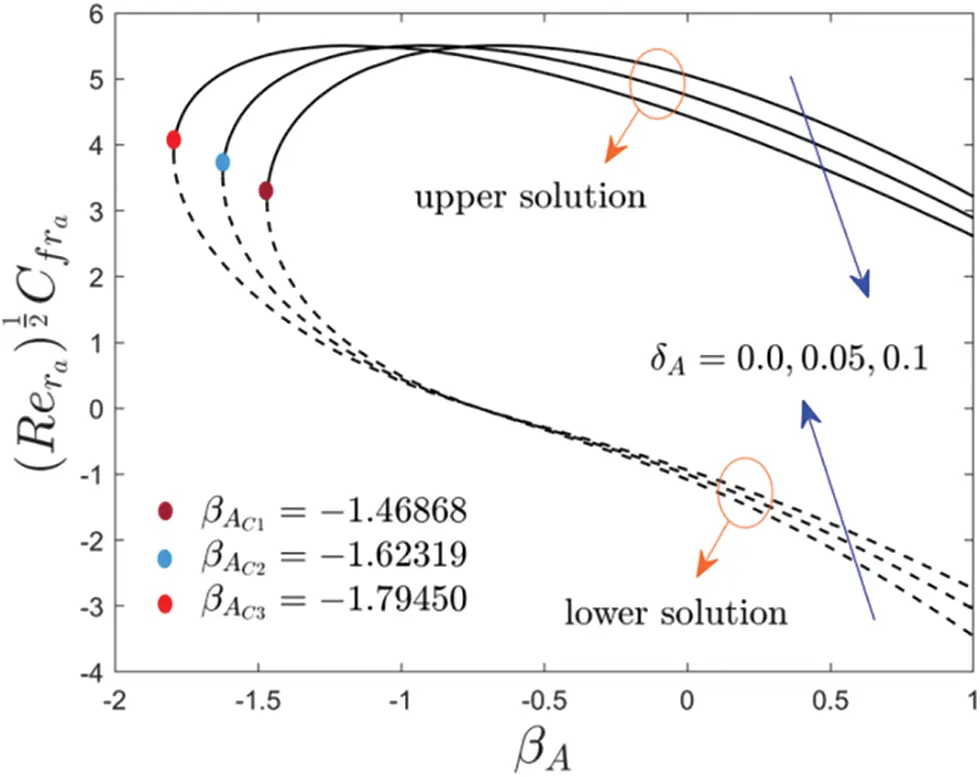Figure 4: Variations of Rera1/2Cfra against βA for dissimilar choices of δA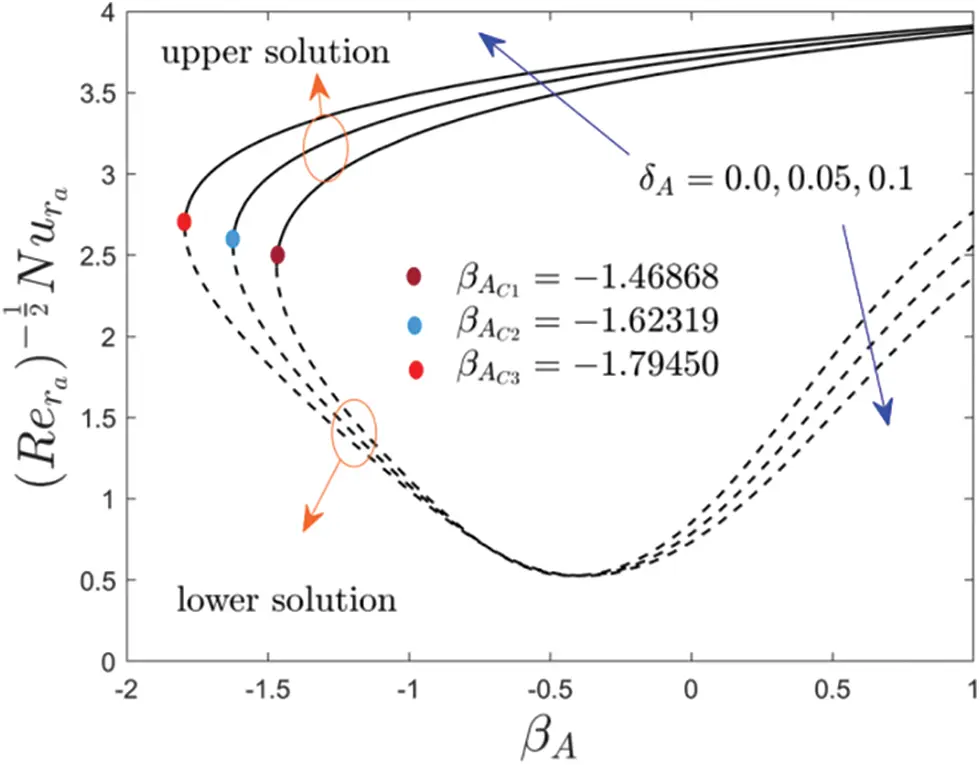Figure 5: Variations of Rera1/2Nura against βA for several values of δAFigure 6: Variations of Rera1/2Nura against βA for dissimilar choices of Nr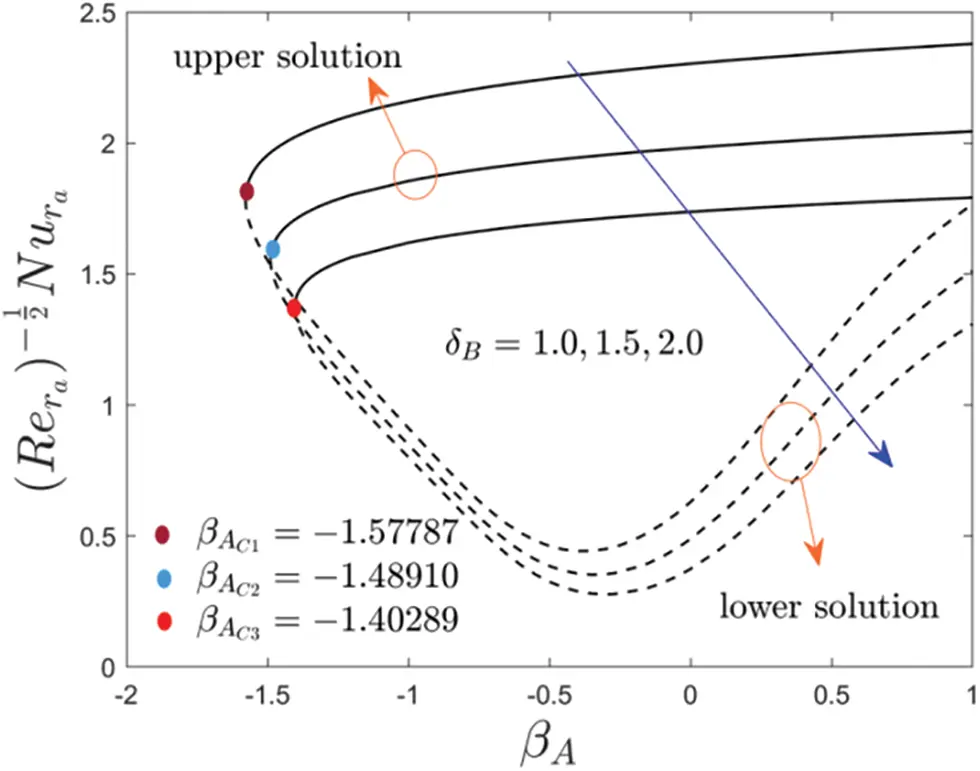Figure 7: Deviations of Rera1/2Nura against βA for several values of δBFigure 8: Variations of S(ξ) against ξ for several values of NrFigure 9: Variations of S(ξ) against ξ for several values of δB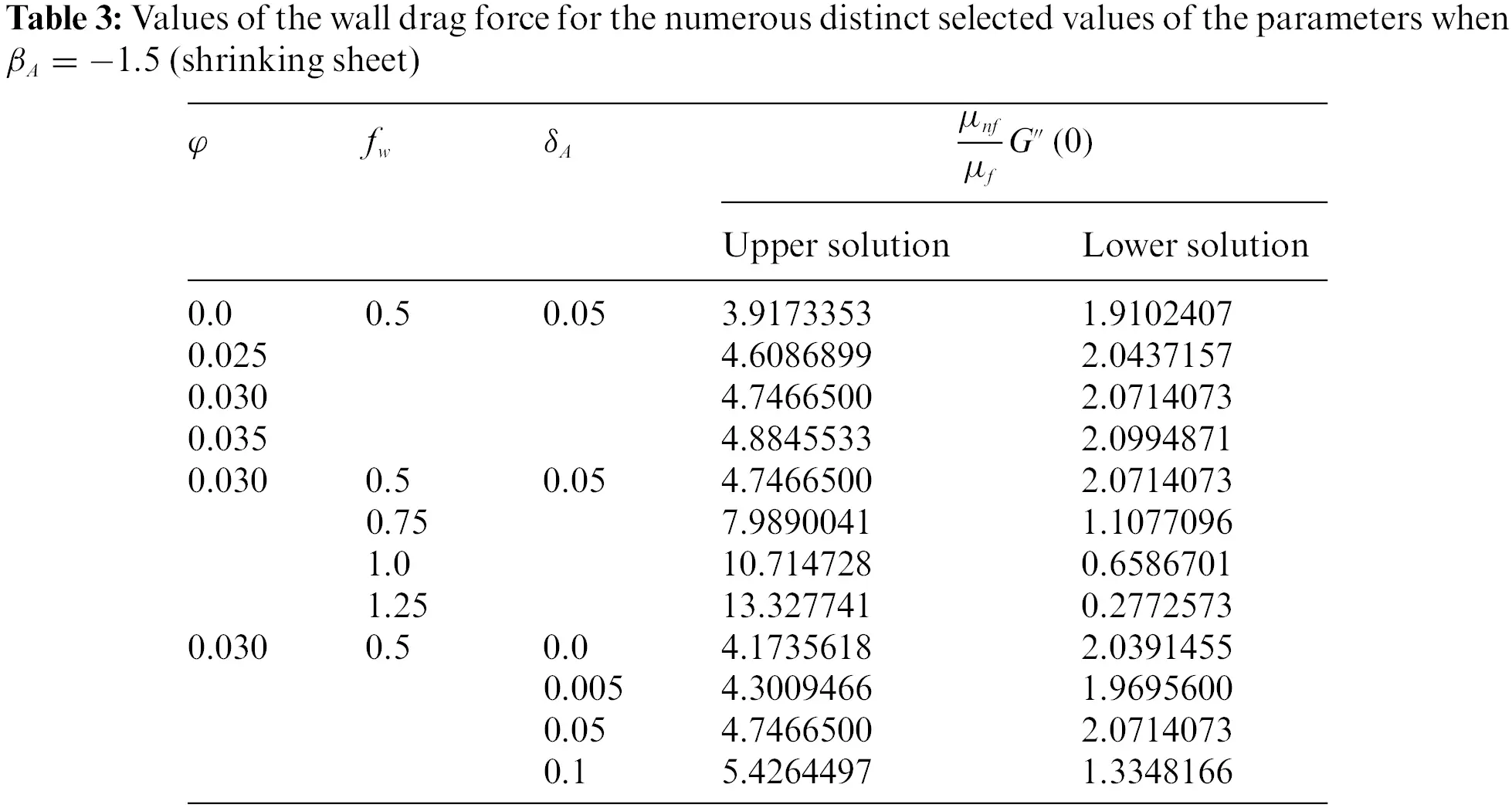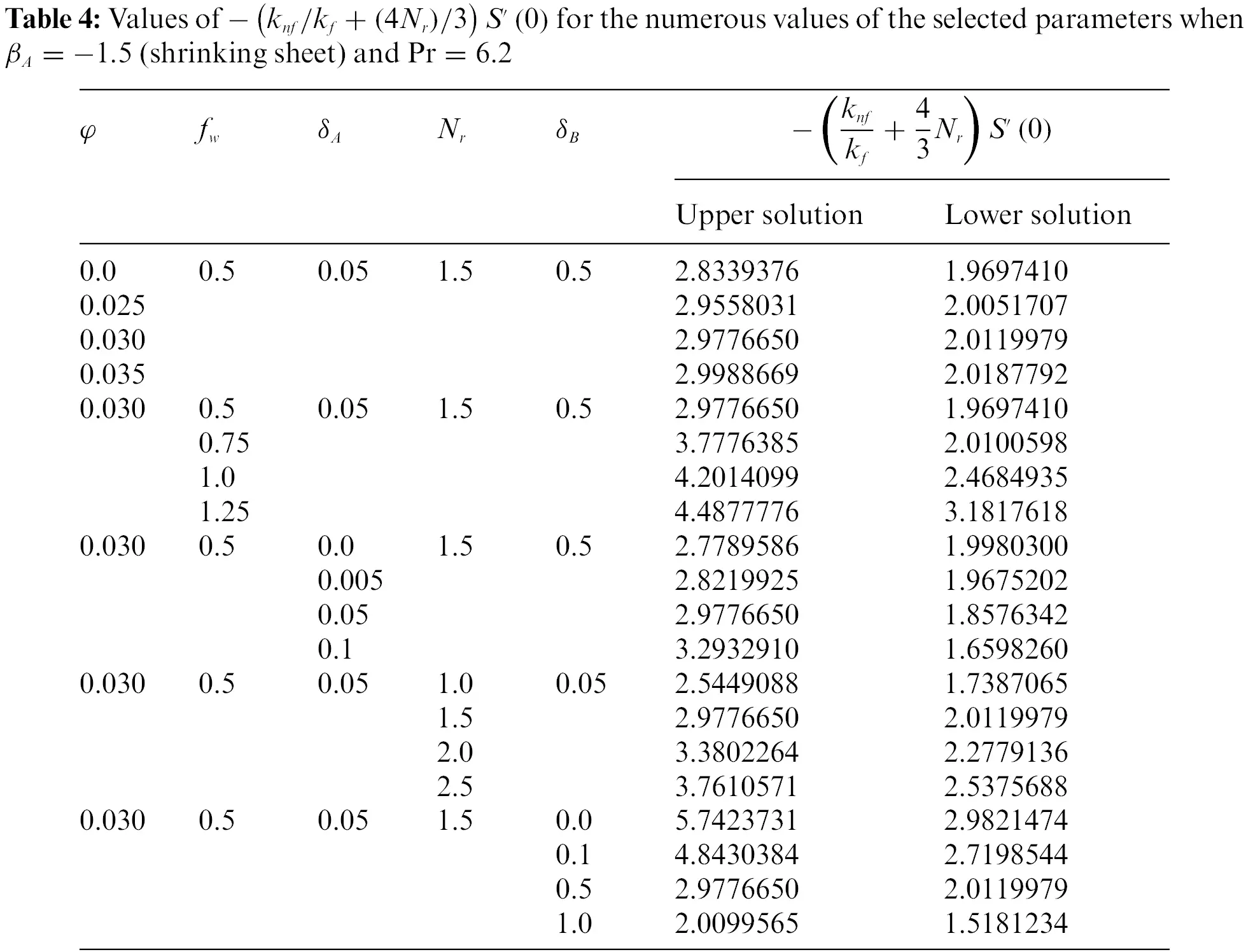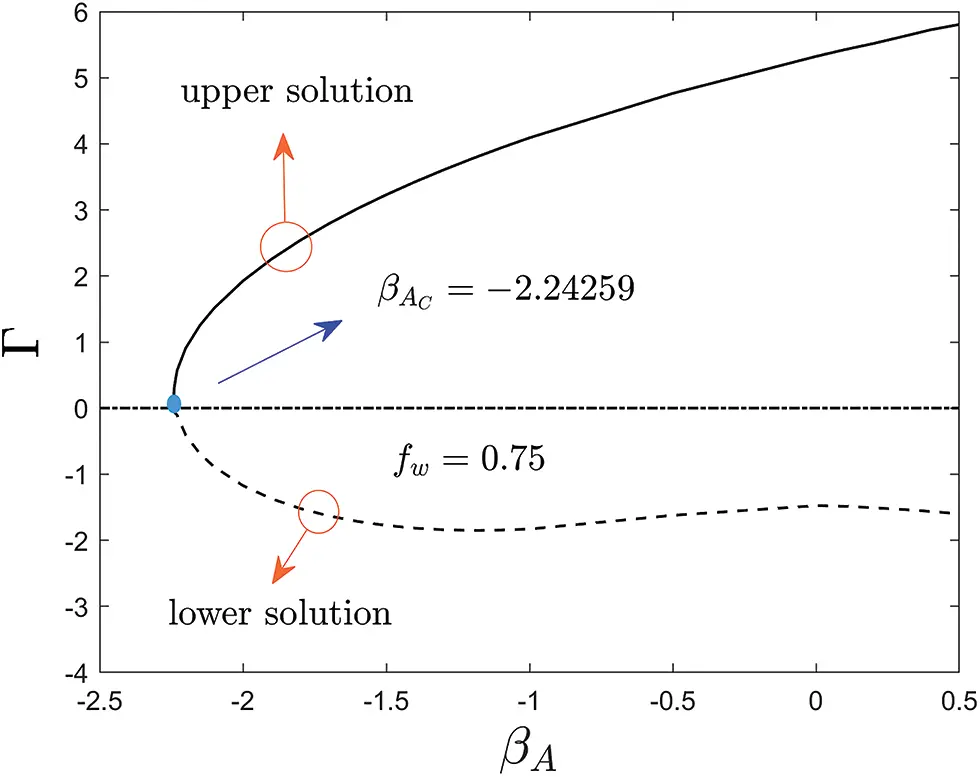Figure 10: Eigenvalues of Γ for several values of βA

Table 3 displays the numerical values of the shear stress for the UB and LB outcomes with the influence of φ, fw and δA when βA=1.5. From the table, it can be seen that the wall drag force upsurges for UB but decreases for the LB with higher values of fw and δA while it is improved in two dissimilar branch results for developed impressions of φ. In addition, the wall drag force is higher for the stable result and lesser for the unstable outcome with fw as compared to the consequence of the factors δA and φ. Alternatively, the numerical values of the heat transfer for the UB and LB results with the impact of φ, fw, δA, Nr and δB when βA=1.5 and Pr=6.2 as highlighted in Table 4. The heat transport phenomenon elevates for UB as well as for LB with the higher impact of fw, Nr and φ while it is shrinkages for UB and LB due to δB. Also, the impressions of δA can boost up the tendency of thermal transport for the stable result but decline for the unstable solution.

Figs. 2 to 7 elucidate the variation of wall drag force Rera1/2Cfra, and heat transfer Rera1/2Nura of the copper water-based nanofluid against βA due to the influence of fw, δA, Nr and δB, respectively. From these figures, it can be seen that two distinct branch solutions exist for the Eqs. (10) to (12) in the range of βA>βAC for both moving disk parameter or stretching/shrinking parameter, a unique solution found when βA=βAC and no solution is obtained when βA<βAC, whereas, βAC corresponds the bifurcation value of βA which the upper and lower solution merge.

Moreover, Figs. 2 and 3 show the inspirations of fw on the wall friction force and heat transfer of the (Cu-water) nanofluid against βA. From the responses, it is observed that the upswing of fw augments the wall drag force for the UBS but declines for the LBS while (knf/kf+(4Nr)/3)S(0) is expressively widened with both (UB and LB) solutions. The existence of the solution domain for the occurrence of multiple (UB and LB) solutions is also increasing with the developed impressions of fw. Physically, an increase in fw causes a flow of nanoparticles towards the moving disk surface, as a result, lowering the velocity, and hence the wall drag coefficient is developing. Therefore, the gap between the UB curves is relatively more as compared to the curves of the LB (see Figs. 2 and 3). For growing values of fw the magnitude of bifurcation values (BVs) get developed. Therefore, the following BVs are found such as −1.62319, −2.24259, and −2.96235 for the respective selected values of fw. This trend recommends that growing values of fw decelerates the separation of boundary layers.

In Figs. 4 and 5, the variations of the multiple (UB and LB) solutions with the change of the velocity slip parameter δA are demonstrated with the wall drag force and heat transfer of the copper water-based nanoparticles, respectively. For growing values of δA, the wall drag force decreases and increases for the UBS in the specific range of βA while it is followed the contrary patterns for the LBS as compared to UBS for higher δA. Physically, the liquid particles and the motion of the disk are different at the surface of the moving disk in the corresponding Maxwell slip BCs phenomena, which devalues the motion of fluid and specially the outcomes drop in the velocity fields. As a result, the friction factor in the radial path upsurges near the bifurcation point. In contrast, the heat transfer enriches for upper branch solution with larger values of δA while the impacts of δA is reversed for the lower solution curves. Moreover, the wall drag force behavior of the solution changes twice (for the UBS as well as for the LBS) at some finite value β0 of βA due to the larger value of δA while for heat transfer it is changed the only single time for the LBS curves. Owing to the improvement of the velocity slip parameter δA, the bifurcation values of the moving disk parameter are −1.46868, −1.62319, and −1.79450, respectively. In this respect, the separation of the boundary layer becomes suspended with the superior value of δA.

The influence of the radiation parameter Nr and temperature slip parameter δB on the heat transfer against βA is revealed in Figs. 6 and 7, respectively. From the outcomes, it is perceived that the rate of heat transfer elevates for both solution branches with Nr but decelerates due to the larger value of δB. Generally, the liquid particles attract more thermal heat by increasing the radiation parameter; as a response, the heat transfer increases (see Fig. 6). In addition, with the selected change value of Nr, the subsequent BVs are found such as −1.62319, −1.76068, and −1.90819, respectively. To see more deeply, the pattern of the BVs decreases with Nr and consequently, the separation of boundary layer shrinkages. Alternatively, the magnitude of the BVs |βAC| reduces for the larger value of δB as presented in Fig. 7. Therefore, the behavior of the solution curves specifies that the boundary layer separation augments with δB.

Figs. 8 and 9 describe the behavior of the typical temperature profiles for the multiple solutions of the copper-water base nanoparticles against the pseudo-similarity variable ξ with Nr and δB, respectively. The temperature profile in Fig. 8 augments for both branches of solution with a rise in Nr. The physical reason is that Nr is the key factor of the improvement of heat transfer. This situation is divulged that the (knf/kf+(4Nr)/3)S(0) enlarges via the asset of augmentation in the value of Nr. It is since an upsurge in Nr shrinkages the ka which consequently drives to appreciation in temperature profiles. Conversely, the typical temperature decelerates for multiple (upper and lower) solutions due to the enhancement in the value of δB (see Fig. 9). The general reason is that the increment in δB lessening the distance between the wall moving surface of the disk and the free-stream temperature which convey less energy or heat to the liquid particles from the moving disk surface, and subsequently lessen the fluid temperature and the temperature profile as well.

Finally, Table 5 displays the eigenvalues Γ against βA for the UB and LB solutions, while the rest of the comprised constraints are taken to be fixed. The data shown in the table are further plotted in the form of a graph (see Fig. 10) which can distinguish the behavior of the upper and lower solution noticeably. This graph is drawn for the positive and negative values of Γ against βA. So, for the obtained smallest positive numerical values of Γ, it is distinguished that eΓΣ0 as time evolves (Σ). In the meantime, for the negative numerical values of Γ, it is observed that eΓΣ0 as time evolves (Σ). Therefore, the UBS is stable and hence physically trustworthy as Σ, while the LBS is not.

6  Conclusions

The influence of Maxwell velocity and Smoluchowski temperature slip conditions on the Agrawal steady axisymmetric SPF of (Cu-water) nanofluid via a moving continuous disk comprising the significant impacts of suction/injection owing to solar energy radiation has been examined. The employment of a similarity transformation for the steady Agrawal nanofluid flow is of particular relevance in this work. The significant outcomes are summarized as follows:

•   The shear stress and heat transfer have been found to show double (UB and LB) solutions over a wide range of moving disk parameter or stretching/shrinking parameter.

•   The shear stress uplifts due to fw in the UB solution and shrinks in the case of LB solution; however, the heat transfer augments due to fw in both cases.

•   The heat transfer for the upper and lower solution branches enhances due to Nr, while it shrinkages with a higher value of δB.

•   The thermal boundary layer thickness of (Cu-water) nanofluid is decayed for both branches owing to the development of δB, while it is strengthened due to the larger value of Nr.

•   The domain of existence of multiple (upper and lower) solutions significantly improves for the larger values of the temperature slip parameter, radiation parameter, velocity slip parameter, and suction parameter.

•   The velocity slip parameter δB has a dramatic impact on the shear stress for the UB and LB solutions while the heat transfer rate is increased and decreased significantly with a higher value of δB for the UB and LB solutions, respectively.

•   The magnitude of the bifurcation or critical values enhances due to enlarging values of Nr, fw and δB, but reduces with the superior value of δB.

•   The boundary layer separation is delayed or broken down with a higher impact of Nr, fw and δB while the separation of the boundary layer is escalated owing to a higher value of δB.

•   Well-disposed to the analysis of stability, it was established that the UB solution is physically stable and trustworthy, while the LB solution is not.

The influence of nanoparticles on the absorption of radiative energy has been of interest for many years in a variety of applications. Nowadays, researchers are interested in the radiation properties of nanoparticles in liquid suspensions, especially for medical and other engineering or technical applications. In addition to the advantages of optical and radiative properties, nanofluids provide other advantages such as improved thermal conductivity and particle stability over micrometer-sized suspensions and the operation of direct-absorbing solar collectors. Nanofluids have been shown to possess improved heat transport properties and higher energy efficiency in a variety of thermal exchange systems for different industrial applications, such as transportation, electronic cooling, military, nuclear energy, aerospace, etc. Nanofluids powered by solar energy are important because they can be used in many heat transfers and other applications such as detergents, solar panels, drying processes, heat exchangers, geothermal and oil recovery, and building construction, etc.

7  Future Work

The future potential investigations of interest are as follows:

•   The current work can be extended by considering the hybrid nanofluids to investigate the Agrawal flow.

•   The time-dependent flow may be considered in future work.

•   The mixed convection flow may be considered.

Acknowledgement: This research was supported by Researchers Supporting Project No. (RSP-2021/33), King Saud University, Riyadh, Saudi Arabia.

Funding Statement: The authors received the financial support from King Saud University, Riyadh, Saudi Arabia through Project No. RSP-2021/33.

Conflicts of Interest: The authors declare that they have no conflicts of interest to report regarding the present study.

## References

1. Angstrom, A. K. (1924). Solar and terrestrial radiation. Quarterly Journal of the Royal Meteorological Society, 50(210), 121-126. [Google Scholar] [CrossRef]
2. Keshavarz, M. M., & Majid, H. (2012). Modeling of turbulent forced convective heat transfer and friction factor in a tube for FeO magnetic nanofluid with computational fluid dynamics. International Communications in Heat and Mass Transfer, 39(8), 1293-1296. [Google Scholar] [CrossRef]
3. Hunt, A. J. (1978). Small particle heat exchangers. Journal of Renewable and Sustainable Energy. Lawrence Berkeley Laboratory Report No. LBL-7841.
4. Lu, L., Liu, Z. H., & Xiao, H. S. (2011). Thermal performance of an open thermosyphon using nanofluids for high-temperature evacuated tubular solar collectors: Part 1: Indoor experiment. Solar Energy, 85(2), 379-387. [Google Scholar] [CrossRef]
5. Kandasamy, R., Muhaimin, I., Khamis, A. B., & Roslan, R. B. (2013). Unsteady Hiemenz flow of Cu-nanofluid over a porous wedge in the presence of thermal stratification due to solar energy radiation: Lie group transformation. International Journal of Thermal Sciences, 65, 196-205. [Google Scholar] [CrossRef]
6. Anbuchezhian, N., Srinivasan, K., Chandrasekaran, K., & Kandasamy, R. (2013). Magneto-hydrodynamic effects on natural convection flow of a nanofluid in the presence of heat source due to solar energy. Meccanica, 48(2), 307-321. [Google Scholar] [CrossRef]
7. Kandasamy, R., Muhaimin, I., & Rosmila, A. K. (2014). The performance evaluation of unsteady MHD non-Darcy nanofluid flow over a porous wedge due to renewable (solar) energy. Renewable Energy, 64, 1-9. [Google Scholar] [CrossRef]
8. Eid, M. R., & Makinde, O. D. (2018). Solar radiation effect on a magneto nanofluid flow in a porous medium with chemically reactive species. International Journal of Chemical Reactor Engineering, 16(9), 20170212. [Google Scholar] [CrossRef]
9. Talebi, M. H., Kalantar, V., Nazari, M. R., & Kargarsharifabad, H. (2019). Experimental investigation of the forced convective heat transfer of hybrid Cu/FeO nanofluids. Journal of Solid and Fluid Mechanics, 8(4), 229-238. [Google Scholar] [CrossRef]
10. Acharya, N. (2020). On the flow patterns and thermal behaviour of hybrid nanofluid flow inside a microchannel in presence of radiative solar energy. Journal of Thermal Analysis and Calorimetry, 141(4), 1425-1442. [Google Scholar] [CrossRef]
11. Asadikia, A., Mirjalily, S. A. A., Nasirizadeh, N., & Kargarsharifabad, H. (2020). Hybrid nanofluid based on CuO nanoparticles and single-walled Carbon nanotubes: Optimization, thermal, and electrical properties. International Journal of Nano Dimension, 11(3), 277-289. [Google Scholar]
12. Asadikia, A., Mirjalily, S. A. A., Nasirizadeh, N., & Kargarsharifabad, H. (2020). Characterization of thermal and electrical properties of hybrid nanofluids prepared with multi-walled carbon nanotubes and FeO nanoparticles. International Communications in Heat and Mass Transfer, 117(4), 104603. [Google Scholar] [CrossRef]
13. Sarkar, A., Kundu, P. K. (2021). Framing the upshot of Hall current on MHD unsteady nanofluid flow from a rotating spherical body in presence of solar radiation. International Journal of Ambient Energy, 1–11. DOI 10.1080/01430750.2021.1965021. [CrossRef]
14. Asadikia, A., Mirjalily, S. A. A., Nasirizadeh, N., & Kargarsharifabad, H. (2021). Experimental study of thermal conductivity of water/ethylene glycol-FeO/SWNCT composite nanofluid and its optimization using neural networks and genetic algorithms. Journal of Nanomaterials, 13(45), 1-14. [Google Scholar]
15. Chamkha, A. J., Armaghani, T., Mansour, M. A., Rashad, A. M., & Kargarsharifabad, H. (2021). MHD convection of an AlO Cu/water hybrid nanofluid in an inclined porous cavity with internal heat generation/absorption. Iranian Journal of Chemistry and Chemical Engineering, 1, 1-10. [Google Scholar] [CrossRef]
16. Shoeibi, S., Kargarsharifabad, H., Rahbar, N., Ahmadi, G., & Safaei, M. R. (2022). Performance evaluation of a solar still using hybrid nanofluid glass cooling-CFD simulation and environmental analysis. Sustainable Energy Technologies and Assessments, 49(4), 101728. [Google Scholar] [CrossRef]
17. Navier, C. L. M. H. (1827). Mémoire sur les lois du mouvement des fluids. Mémoires de l’Académie des Sciences, 6, 389-391. [Google Scholar]
18. Maxwell, J. C. (1878). On stresses in rarefied gases arising from inequalities of temperature. Proceedings of the Royal Society of London, 27(185–189), 304-308. [Google Scholar] [CrossRef]
19. Smoluchowski, M. V. (1898). Ueber Wärmeleitung in verdünnten Gasen. Annalen der Physik, 300(1), 101-130. [Google Scholar] [CrossRef]
20. Ramya, D., Raju, R. S., Anand, J. A., & Chamkha, A. J. (2018). Effects of velocity and thermal wall slip on magnetohydrodynamics (MHD) boundary layer viscous flow and heat transfer of a nanofluid over a non-linearly-stretching sheet: A numerical study. Propulsion and Power Research, 7(2), 182-195. [Google Scholar] [CrossRef]
21. Khashi, N. S., Arifin, N. M., Pop, I., Nazar, R., & Hafidzuddin, E. H. (2020). Three-dimensional hybrid nanofluid flow and heat transfer past a permeable stretching/shrinking sheet with velocity slip and convective condition. Chinese Journal of Physics, 66(4), 157-171. [Google Scholar] [CrossRef]
22. Jünemann, T., Pleskun, H., & Brümmer, A. (2021). Maxwell velocity slip and Smoluchowski temperature jump boundary condition for ANSYS CFX. IOP Conference Series: Materials Science and Engineering, 1180(1), 012037. [Google Scholar] [CrossRef]
23. Sajid, T., Jamshed, W., Shahzad, F., Akgül, E. K., & Nisar, K. S. (2022). Impact of gold nanoparticles along with Maxwell velocity and Smoluchowski temperature slip boundary conditions on fluid flow: Sutterby model. Chinese Journal of Physics, 77(2), 1387-1404. [Google Scholar] [CrossRef]
24. Hiemenz, K. (1911). Die Grenzschicht an einem in den gleichformingen Flussigkeitsstrom eingetauchten graden Kreiszylinder. Dinglers Polytechnisches Journal, 326, 321-324. [Google Scholar]
25. Homann, F. (1936). Der Einfluss grosser Zahigkeit bei der Stromung um den Zylinder und um die Kugel. Journal of Applied Mathematics and Mechanics, 16(3), 153-164. [Google Scholar] [CrossRef]
26. Howarth, L. (1951). The boundary layer in three dimensional flow--Part II, the flow near a stagnation point. Philosophical Magazine and Journal of Science, 42(335), 1433-1440. [Google Scholar] [CrossRef]
27. Davey, A. (1961). Boundary layer flow at a saddle point of attachment. Journal of Fluid Mechanics, 10(4), 593-610. [Google Scholar] [CrossRef]
28. Agrawal, H. L. (1957). A new exact solution of the equations of viscous motion with axial symmetry. The Quarterly Journal of Mechanics and Applied Mathematics, 10(1), 42-44. [Google Scholar] [CrossRef]
29. Weidman, P. (2015). Axisymmetric rotational stagnation-point flow impinging on a rotating disk. Zeitschrift für Angewandte Mathematik und Physics, 66(6), 3425-3431. [Google Scholar] [CrossRef]
30. Weidman, P. (2016). Axisymmetric rotational stagnation point flow impinging on a flat liquid surface. European Journal of Mechanics-B/Fluids, 56, 188-191. [Google Scholar] [CrossRef]
31. Weidman, P. (2016). Axisymmetric rotational stagnation point flow impinging on a radially stretching sheet. International Journal of Non-Linear Mechanics, 82, 1-5. [Google Scholar] [CrossRef]
32. Roşca, N. C., & Pop, I. (2017). Axisymmetric rotational stagnation point flow impinging radially a permeable stretching/shrinking surface in a nanofluid using Tiwari and Das model. Scientific Reports, 7(1), 40299. [Google Scholar] [CrossRef]
33. Fathalah, K. A., & Elsayed, M. M. (1980). Natural convection due to solar radiation over a non-absorbing plate with and without heat losses. International Journal of Heat and Fluid Flow, 2(1), 41-45. [Google Scholar] [CrossRef]
34. Acharya, N., Das, K., & Kundu, P. K. (2016). The squeezing flow of Cu-water and Cu-kerosene nanofluids between two parallel plates. Alexandria Engineering Journal, 55(2), 1177-1186. [Google Scholar] [CrossRef]
35. Hayat, T., Qayyum, S., Khan, M. I., & Alsaedi, A. (2018). Entropy generation in magnetohydrodynamic radiative flow due to rotating disk in presence of viscous dissipation and Joule heating. Physics of Fluids, 30(1), 017101. [Google Scholar] [CrossRef]
36. Merkin, J. H. (1986). On dual solutions occurring in mixed convection in a porous medium. Journal of Engineering Mathematics, 20(2), 171-179. [Google Scholar] [CrossRef]
37. Weidman, P. D., Kubitschek, D. G., & Davis, A. M. J. (2006). The effect of transpiration on self-similar boundary layer flow over moving surfaces. International Journal of Engineering Science, 44(11–12), 730-737. [Google Scholar] [CrossRef]
38. Shampine, L. F., & Thompson, S. (2001). Solving DDEs in MATLAB. Applied Numerical Mathematics, 37(4), 441-458. [Google Scholar] [CrossRef]
39. Khan, U., Waini, I., Ishak, A., & Pop, I. (2021). Unsteady hybrid nanofluid flow over a radially permeable shrinking/stretching surface. Journal of Molecular Liquids, 331(3), 115752. [Google Scholar] [CrossRef]

Khan, U., Zaib, A., Ishak, A., Waini, I., Sherif, E. M. et al. (2023). Stability Scrutinization of Agrawal Axisymmetric Flow of Nanofluid through a Permeable Moving Disk Due to Renewable Solar Radiation with Smoluchowski Temperature and Maxwell Velocity Slip Boundary Conditions. CMES-Computer Modeling in Engineering & Sciences, 134(2), 1371–1392.This work is licensed under a Creative Commons Attribution 4.0 International License , which permits unrestricted use, distribution, and reproduction in any medium, provided the original work is properly cited.

View

Like

## Related articles

• Martínez-Rubin de Celis E, E Rivas-Robles,...
• Manoj Kr. Triveni, Rajsekhar Panua
• Fatiha Bentarzi, Amina Mataoui...
• H. Azoui, D. Bahloul, N. Soltani
• G. Venkata Ramana Reddy, Y. Hari...